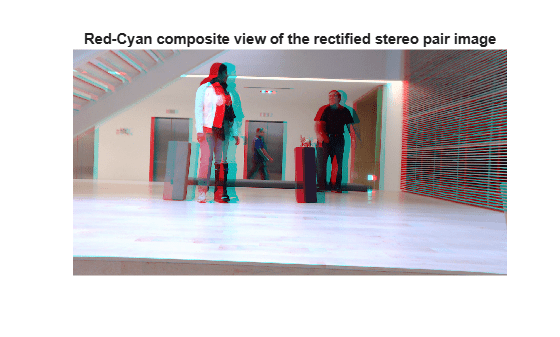# disparitySGM

Compute disparity map through semi-global matching

## Syntax

``disparityMap = disparitySGM(I1,I2)``
``disparityMap = disparitySGM(I1,I2,Name,Value)``

## Description

example

````disparityMap = disparitySGM(I1,I2)` computes disparity map from a pair of rectified stereo images `I1` and `I2`, by using semi-global matching (SGM) method. To know more about rectifying stereo images, see Image Rectification.```
````disparityMap = disparitySGM(I1,I2,Name,Value)` specifies options using one or more name-value pair arguments.```

## Examples

collapse all

Load a rectified stereo pair image.

```I1 = imread('rectified_left.png'); I2 = imread('rectified_right.png');```

Create the stereo anaglyph of the rectified stereo pair image and display it. You can view the image in 3-D by using red-cyan stereo glasses.

```A = stereoAnaglyph(I1,I2); figure imshow(A) title('Red-Cyan composite view of the rectified stereo pair image')```Convert the rectified input color images to grayscale images.

```J1 = rgb2gray(I1); J2 = rgb2gray(I2);```

Compute the disparity map through semi-global matching. Specify the range of disparity as [0, 48], and the minimum value of uniqueness as 20.

```disparityRange = [0 48]; disparityMap = disparitySGM(J1,J2,'DisparityRange',disparityRange,'UniquenessThreshold',20);```

Display the disparity map. Set the display range to the same value as the disparity range.

```figure imshow(disparityMap,disparityRange) title('Disparity Map') colormap jet colorbar```## Input Arguments

collapse all

Input image referenced as `I1` corresponding to camera 1, specified as a 2-D grayscale image or a `gpuArray` (Parallel Computing Toolbox) object. The function uses this image as the reference image for computing the disparity map. The input images `I1` and `I2` must be real, finite, and nonsparse. Also, `I1` and `I2` must be of the same size and same data type.

Data Types: `single` | `double` | `int16` | `uint8` | `uint16`

Input image referenced as `I2` corresponding to camera 2, specified as a 2-D grayscale image or a `gpuArray` (Parallel Computing Toolbox) object. The input images `I1` and `I2` must be real, finite, and nonsparse. `I1` and `I2` must be of the same size and same data type.

Data Types: `single` | `double` | `int16` | `uint8` | `uint16`

### Name-Value Arguments

Specify optional pairs of arguments as `Name1=Value1,...,NameN=ValueN`, where `Name` is the argument name and `Value` is the corresponding value. Name-value arguments must appear after other arguments, but the order of the pairs does not matter.

Before R2021a, use commas to separate each name and value, and enclose `Name` in quotes.

Example: `disparitySGM(I1,I2,'DisparityRange',[0 64])`

Range of disparity, specified as the comma-separated pair consisting of `'DisparityRange'` and a two-element vector of the form [MinDisparity MaxDisparity]. MinDisparity is the minimum disparity and MaxDisparity is the maximum disparity.

For input images of width N, the MinDisparity and MaxDisparity must be integers in the range (–N, N). The difference between the MaxDisparity and MinDisparity values must be divisible by 8 and must be less than or equal to 128.

The default value for the range of disparity is `[0 128]`. For more information on choosing the range of disparity, see Choosing Range of Disparity.

Data Types: `integers`

Minimum value of uniqueness, specified as the comma-separated pair consisting of `'UniquenessThreshold'` and a nonnegative integer.

The function marks the estimated disparity value K for a pixel as unreliable, if:

v < V×(1+0.01×`UniquenessThreshold`),

where V is the Hamming distance corresponding to the disparity value K. v is the smallest Hamming distance value over the whole disparity range, excluding K, K–1, and K+1.

Increasing the value of `UniquenessThreshold` results in disparity values for more pixels being marked as unreliable. To disable the use of uniqueness threshold, set this value to 0.

## Output Arguments

collapse all

Disparity map for rectified stereo pair image, returned as a 2-D grayscale image or a `gpuArray` object. The function returns the disparity map with the same size as input images `I1` and `I2`. Each value in this output refers to the displacement between conjugate pixels in the stereo pair image. For details about computing the disparity map, see Computing Disparity Map Using Semi-Global Matching.

Data Types: `single`

collapse all

### Image Rectification

The input images `I1` and `I2` must be rectified before computing the disparity map. The rectification ensures that the corresponding points in the stereo pair image are on the same rows. You can rectify the input stereo pair image by using the `rectifyStereoImages` function. The reference image must be the same for rectification and disparity map computation.

## Algorithms

collapse all

### Choosing Range of Disparity

The range of disparity must be chosen to cover the minimum and the maximum amount of horizontal shift between the corresponding pixels in the rectified stereo pair image. You can determine the approximate horizontal shift values from the stereo anaglyph of the stereo pair image. Compute the stereo anaglyph of the rectified images by using the `stereoAnaglyph` function. Display the stereo anaglyph in the Image Viewer app. To measure the amount of horizontal shift between the corresponding points in the stereo pair image, select Measure Distance from the Tools menu in Image Viewer. Choose the minimum and maximum disparity values for the range of disparity based on this measurement.

For example, this figure displays the stereo anaglyph of a rectified stereo pair image and the horizontal shift values measured between the corresponding points in the stereo pair image. The minimum and maximum shift values are computed as 8 and 31 respectively. Based on these values, the range of disparity can be chosen as [0, 48].### Computing Disparity Map Using Semi-Global Matching

1. Compute Census transform of the rectified stereo pair image.

2. Compute Hamming distance between pixels in the census-transformed image to obtain the matching cost matrix.

3. Compute the pixel-wise disparity from matching cost matrix by using the semi-global matching method given in .

4. Optionally, mark the pixels for unreliability based on the `UniquenessThreshold` name-value pair. The function sets the disparity values of the unreliable pixels to `NaN`.

 Hirschmuller, H. "Accurate and Efficient Stereo Processing by Semi-Global Matching and Mutual Information." In Proceedings of the IEEE Conference on Computer Vision and Pattern Recognition (CVPR), pp. 807-814. San Diego, CA: IEEE, 2005.